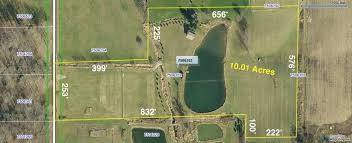# How Many Square Feet Is .22 Acres?

0.22 acres converts to 9583.2 square feet.An acre is the same as 43,560 square feet (or, to put it another way, 208.71 feet by 208.71 feet). As 0.22 is roughly one-fifth as a fraction, you can make a rough estimate in your head along the lines of dividing 43,560 by five, to give 8712 square feet, knowing you would be slightly under the actual total.

What is the Formula Required to Convert Acres to Feet?

If you find yourself without internet access or a calculator, and need to known how many square feet are in a given number of acres, who can follow this formula:

Acres x 43,560 = square feet.

In this instance:

0.22 x 43,560 = 9583.2 square feet.

If you wanted to converted this amount to metric, remember that a square foot is roughly 0.092 square meters, so divide the total by ten for a rough estimate.

If you're still confused, this film should help clear things up:

thanked the writer.